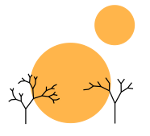# The Halting Problem

Rimu Shuang. "Untitled Photo". Nov 5, 2013. Under a Creative Commons 3.0 Attribution License.

### math, model_theory

I was recently talking with a friend about computability theory and he mentioned how he was studying the halting problem in his computer science class. Of course the essential content remained the same, but the terminology was curiously very different. I tried to explain how the halting problem to him as mathematicians see it, but I did a very terrible job. Here’s my attempt to make amends.

So what is the halting problem as normally analyzed by mathematicians? In the interest of brevity, I’m going to gloss over the definition of a Turing program right now, but essentially it is a program which represents some subset of the partial functions on the natural numbers. We define a Turing program as a finite set of quintuples (the quintuples themselves are not really important, all we really care is that there is some finite way of expressing a Turing program). Then we have an enumeration of the partial functions which are representable by Turing programs (i.e. partial computable functions or p.c. functions) by storing the Turing program as a number1. Let us denote this enumeration as {φx ∣ x ∈ ω} where φx is a partial function (i.e. it may be undefined for certain inputs).

Importantly this enumeration is effective2, which means that I can pass computably from a Turing program to the number that represents it. This allows us later to talk sensibly of constructing a computable function that takes its own number as input.

As a matter of notation, because φx is a partial function, we need notation for when an element is in the domain of φx and when it is not. In particular, φx(n)↓ denotes that φx(n) is defined (i.e. “φx converges on n”) and φx(n)↑ denotes that φx(n) is not defined (i.e. “φx diverges on n).

Thus, the halting problem, like most problems in computability theory, can be phrased as a set membership question. In particular it asks,

Is the set {(x,y) ∈ ω2 ∣ x ∈ Wy} computable?3

Alternatively, given some injective function f : ω2 → ω, is K0 = {f(x,y) ∈ ω ∣ x ∈ Wy} computable?

The proof that this is not computable is relatively straightforward now that we have the relevant terminology nailed down.

Before we examine the halting problem, let us first examine the problem of finding a computable characteristic function for the set K where K = {x ∣ φx(x) ↓ }. Note that K reduces to the halting problem, i.e. if we had a solution to the halting problem, we would have a solution to K. However, we will show that in fact K is not computable, which implies that the halting problem must not be computable then (otherwise a solution to the halting problem would imply a solution to K).

To begin with let us assume that K is in fact computable and therefore has a characteristic function χK = φn. Then take the function ψ where

ψ(x) = φn(x) + 1

Clearly if φ is computable, so is ψ (for any acceptable meaning of computable). However, by construction x(ψ(x)≠φn(x)) and this in turn implies that x(ψ(x)≠φx(x)) and hence there is no index m for which φm = ψ. This means then that ψ is not included in our collection of computable functions and hence φn too must be not computable, a contradiction which implies that K is not computable4.

This means the halting problem is not computable. Tada!

1. The easiest way of noting that any Turing program can be represented by a number is that any computer program ultimately is a binary string and a binary string encodes a number. More specifically, in mathematics, we can use an injective function from ωn → ω. For convenience, a bijective function would probably be best, but nothing suffers except the verbosity of some proofs from using just an injective function.↩︎

2. Effective is basically a synonym for computable. Often it is used to mean that an algorithm exists for a given problem, but the problem itself may be cumbersome to translate into an equivalent formulation on the natural numbers.↩︎

3. A set S is defined to be computable if its characteristic function, i.e. the function χS where

$\chi _S (x) = \begin{cases} 1 \text{ if$x \in S$}\\ 0 \text{ otherwise} \end{cases}$

, is computable.↩︎

4. Mathematics is generally more concerned with K than K0 (i.e. the simplification of the halting problem we have made rather than the halting problem itself). This is mainly because K is easier to work with. For all purposes in computability theory, however, it turns out that K and K0 are both equivalent even if it may seem that K is “weaker” than K0.↩︎